# Case-sensitive SUMIF and SUMIFS in Excel and Google Sheets

In this tutorial, you will learn how to make a case-sensitive SUMIF or SUMIFS formula in Excel and Google Sheets.

The SUMIF and SUMIFS functions are available in both Microsoft Excel and Google Sheets. And in both applications, they are case-insensitive by nature. To conditionally sum cells treating lowercase and uppercase letters as different characters, you'll have to come up with something else.

## Is SUMIF / SUMIFS case sensitive?

No, it is not. In both Excel and Google Sheets, neither SUMIF nor SUMIFS can recognize the letter case. To make sure of this, please consider this simple example:

Supposing you have a list of id's in column A, where uppercase and lowercase letters denote different items. The corresponding sales figures are in column B. The goal is to get a sum of sales for a specific item, say A-01.

With the target id in E1, we construct this classic SUMIF formula:

`=SUMIF(A2:A6, E1, B2:B6)`

And get an absolutely wrong result :(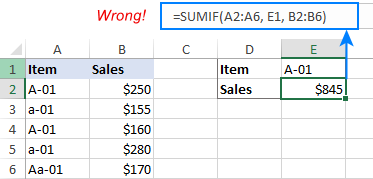## Case-sensitive Sum If formula in Excel

To sum cells with one condition considering the letter case, you can use the SUMPRODUCT and EXACT functions together:

SUMPRODUCT(--(EXACT(criteria, range)), sum_range)

With the target id in E1(criteria), the items list in A2:A10 (range), and sales numbers in B2:B10 (sum_range), the formulas take these forms:

`=SUMPRODUCT(--(EXACT(E1, A2:A10)), B2:B10)`

If needed, you can "hardcode" the criteria directly in the formula:

`=SUMPRODUCT(--(EXACT("A-01", A2:A10)), B2:B10)`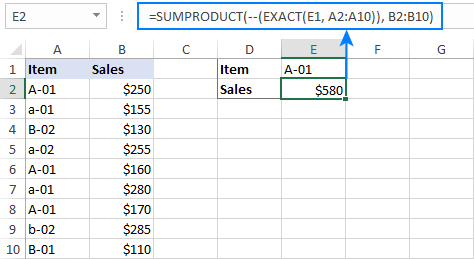How this formula works:

At the heart of the formula, the EXACT function compares the target item (E1) against each item in the list and returns TRUE if the compared values are exactly the same including the text case, FALSE otherwise:

`{TRUE;FALSE;FALSE;FALSE;TRUE;FALSE;TRUE;FALSE;FALSE}`

A double unary operator (--) converts TRUE and FALSE into 1 and 0, respectively:

`{1;0;0;0;1;0;1;0;0}`

The SUMPRODUCT function multiplies the elements of the above array by the corresponding items in B2:B10:

`SUMPRODUCT({1;0;0;0;1;0;1;0;0}, {250;155;130;255;160;280;170;285;110})`

And because multiplying by 0 gives zero, only the items for which EXACT returned TRUE survive:

`SUMPRODUCT({250;0;0;0;160;0;170;0;0})`

Finally, SUMPRODUCT adds up the products and outputs the sum.

## Case-sensitive Sum Ifs in Excel (with multiple criteria)

In case you are looking for a case-sensitive SUMIFS formula with two or more criteria, you can emulate this behavior by defining an additional range/criteria pair in another EXACT function:

SUMPRODUCT(--(EXACT(criteria1, range1)), --(EXACT(criteria2, range2)), sum_range)

For example, to sum sales for a particular item (F1) in a given region (F2), the formula goes as follows:

`=SUMPRODUCT(--(EXACT(F1, A2:A10)), --(EXACT(F2, B2:B10)), C2:C10)`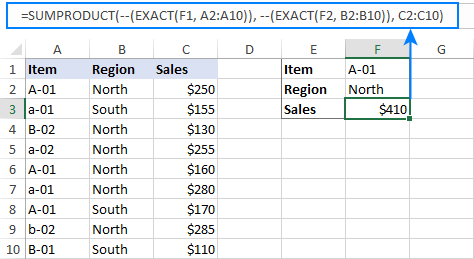## Case-sensitive 'Sum If Cell Contains' formula in Excel

In situation when you need to add up values in one column if a cell in another column contains certain text as part of the cell's content, then use the SUMPRODUCT function together with FIND:

SUMPRODUCT(--(ISNUMBER(FIND(criteria, range))), sum_range)

For example, to sum sales for the item in E1 (which can match an entire cell in A2:A10 or be just part of the text string), the formula is:

`=SUMPRODUCT(--(ISNUMBER(FIND(E1, A2:A10))), B2:B10)`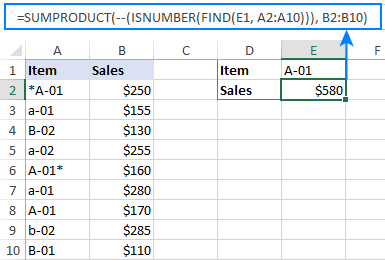To sum cells based on multiple conditions, add one more ISNUMBER/FIND combo:

SUMPRODUCT(--(ISNUMBER(FIND(criteria1, range1))), --(ISNUMBER(FIND(criteria2, range2))), sum_range)

For instance, to get a sum of sales with two conditions (item id in F1 and region in F2), the formula is:

`=SUMPRODUCT(--(ISNUMBER(FIND(F1, A2:A10))), --(ISNUMBER(FIND(F2, B2:B10))), C2:C10)`

Please note that the formula adds up sales for a specified item in any "north" region, such as North, North-East, or North-West.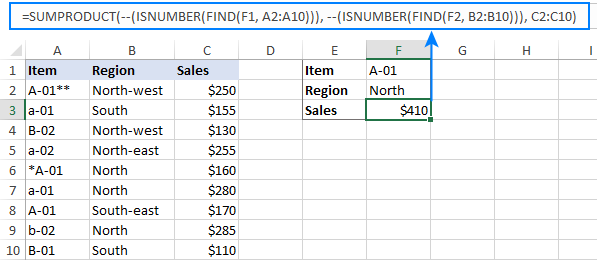How this formula works:

Here, we use the case-sensitive FIND function to search for the target item (E1). When the item is found, the function returns its relative position in the source string, otherwise a #VALUE error.

`{2;#VALUE!;#VALUE!;#VALUE!;1;#VALUE!;1;#VALUE!;#VALUE!}`

The ISNUMBER function converts any number to TRUE and error values to FALSE:

`{TRUE;FALSE;FALSE;FALSE;TRUE;FALSE;TRUE;FALSE;FALSE}`

Next, you do "double negation" (--) to coerce the logical values into 1 and 0:

`{1;0;0;0;1;0;1;0;0}`

Finally, the SUMPRODUCT function multiplies the elements of the two arrays and outputs the result:

`SUMPRODUCT({1;0;0;0;1;0;1;0;0}, {250;155;130;255;160;280;170;285;110})`

If your formula processes multiple conditions, then SUMPRODUCT will multiply the elements of three or more arrays. In our case, it is:

`=SUMPRODUCT({1;0;0;0;1;0;1;0;0}, {1;0;1;1;1;1;0;1;0}, {250;155;130;255;160;280;170;285;110})`

## SUMIF case-sensitive in Google Sheets

The case-sensitive Sum If formulas that we built for Excel will also work in Google Sheets. Additionally, you can get the Google Sheet's SUMIF function itself to distinguish between uppercase and lowercase characters. Here's how:

SUMIF(ArrayFormula(EXACT(criterion, range)), TRUE, sum_range)

Or

SUMIF(ArrayFormula(FIND(criterion, range)), 1, sum_range)

For our sample dataset, the real formulas look as follows:

`=SUMIF(ArrayFormula(EXACT(E1, A2:A10)), TRUE, B2:B10)`

`=SUMIF(ArrayFormula(FIND(E1, A2:A10)), 1, B2:B10)`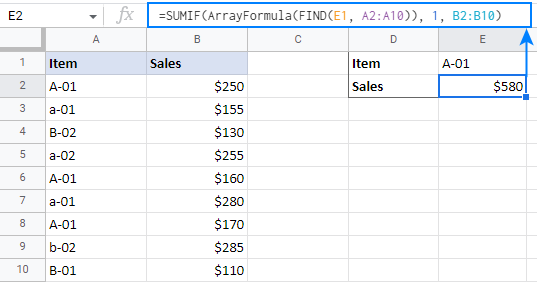How SUMIF/EXACT formula works:

To make the Google Sheets SUMIF(range, criterion, [sum_range]) function recognize the letter case, we use the following formula for the range argument:

`ArrayFormula(EXACT(E1, A2:A10))`

ArrayFormula forces EXACT to compare the value in E1 against each value in A2:A10. If an exact match is found, the formula returns TRUE, otherwise – FALSE.

In the range of TRUE and FALSE values, SUMIF searches for TRUE and adds up the corresponding values in B2:B10. That's all!

How SUMIF/FIND formula works:

Here, we use the combination of ArrayFormula and FIND to look for the target value (E1) in the range A2:A10:

`ArrayFormula(FIND(E1, A2:A10))`

If used separately, the FIND function will stop searching after finding the first match.

Wherever the target value is found, the formula returns 1 (which is its relative position in the search string). For cells where the value is not found, a #VALUE! error is returned.

Next, you use the number 1 as SUMIF's criteria and have the job done :)

## SUMIFS case-sensitive in Google Sheets

To sum cells with multiple conditions in Google Sheets, you can use either case-sensitive SUMPRODUCT formulas discussed in Excel's part of our tutorial or Google Sheet's SUMIFS in combination with EXACT or FIND:

SUMIFS(sum_range, ArrayFormula(EXACT(criterion1, range1)), TRUE, ArrayFormula(EXACT(criterion2, range2)), TRUE)

Or

SUMIFS(sum_range, ArrayFormula(FIND(criterion1, range1)), 1, ArrayFormula(FIND(criterion2, range2)), 1)

As you see, the logic is the same as with the SUMIF function. The difference is that you use two or more range/criterion pairs.

For example, to sum sales for the item in F1 and the region in F2, the formulas are:

`=SUMIFS(C2:C10, ArrayFormula(EXACT(F1, A2:A10)), TRUE, ArrayFormula(EXACT(F2, B2:B10)), TRUE)`

`=SUMIFS(C2:C10, ArrayFormula(FIND(F1, A2:A10)), 1, ArrayFormula(FIND(F2, B2:B10)), 1)`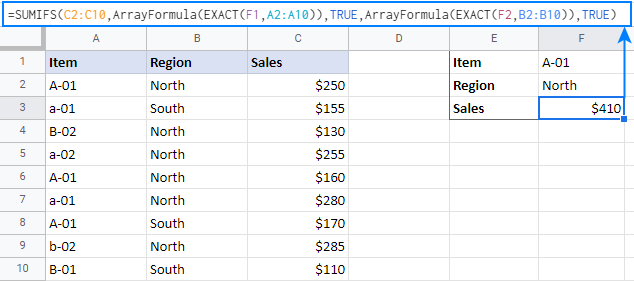This is how you can create a case-sensitive SUMIF or SUMIFS formula in Excel and Google Sheets. I thank you for reading and hope to see you on our blog next week!

## Practice workbooks

Case-sensitive SUMIF / SUMIFS in Excel (.xlsx file)
Case-sensitive SUMIF / SUMIFS in Google Sheets (online sheet)

## You may also be interested in:

### 4 comments to "Case-sensitive SUMIF and SUMIFS in Excel and Google Sheets"

1.Jugal Kishor says:

Case Sensitive Sum
Question:- I want to sum of total ,Name only Binod(only Binod) not binod, Region West?

Names Region Total Output 110
Binod west

Names Region Calls
Binod West 20
binod East 30
Binod West 60
Binod East 20
binod East 60
binod West 50
Binod West 30

•Alexander Trifuntov (Ablebits Team) says:

Hello!

You can find the detailed info on the case-sensitive SUMIFS in Excel and Google Sheets in the above post.

Or feel free to try the following formula:
=SUMPRODUCT(--(EXACT(A2:A8,"Binod")),--(B2:B8="West"),C2:C8)

2.Shailendra Singh patel says:

My issue is not covered in any blog. Please refer mail sent to you

•Natalia Sharashova (Ablebits Team) says:

Hello Shailendra,